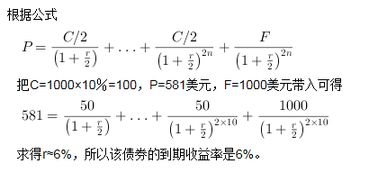# 按面值出售的债券息票率为

## 3.关于债券的息票利率和到期收益率

eg 某债券期限5年，面值1000元，年息60元，市场售价1020元，则该债券的到期收益率（）

1020=60/(1+r%)^1+60/(1+r%)^2+60/(1+r%)^3+60/(1+r%)^4+60/(1+r%)^5+1000/(1+r%)^5

r=5.531256572%